# Following mechanism has been proposed for a reaction, 2A+B $\to$D+E A+B$\to$ C+D    ...(Slow) A+ C$\to$ E         ...(Fast) The rate law expression for the reaction is: 1. r = K[A]2[B] 2. r=K[A][B] 3. r= K[A]2 4. r= K[A][C]

Subtopic:  Definition, Rate Constant, Rate Law |
To view explanation, please take trial in the course below.
NEET 2023 - Target Batch - Aryan Raj Singh
Please attempt this question first.
Launched MCQ Practice Books

Prefer Books for Question Practice? Get NEETprep's Unique MCQ Books with Online Video/Text Solutions via Telegram Bot

In gaseous reactions important for the understanding of the upper atmosphere H2O and O react bimolecularly to form two OH radicals. $∆$H for this reaction is 72kJ at 500 K and Ea is 77 kJ mol-1, then Ea for the bimolecular recombination of two OH radicals to form H2O and O is:

1. 3 kJ mol-1

2. 4 kJ mol-1

3. 5 kJ mol-1

4. 7 kJ mol-1

Subtopic:  Arrhenius Equation |
To view explanation, please take trial in the course below.
NEET 2023 - Target Batch - Aryan Raj Singh
Please attempt this question first.
Launched MCQ Practice Books

Prefer Books for Question Practice? Get NEETprep's Unique MCQ Books with Online Video/Text Solutions via Telegram Bot

For a reaction A Product, rate law is $-\frac{d\left[A\right]}{dt}=K\left[A{\right]}_{0}$. The concentration of A left after time t when $t=\frac{1}{K}$ is:

1. $\frac{\left[A{\right]}_{0}}{e}$

2.

3. $\frac{\left[A{\right]}_{0}}{{e}^{2}}$

4. $\frac{1}{\left[A{\right]}_{0}}$

Subtopic:  First Order Reaction Kinetics |
To view explanation, please take trial in the course below.
NEET 2023 - Target Batch - Aryan Raj Singh
Please attempt this question first.
Launched MCQ Practice Books

Prefer Books for Question Practice? Get NEETprep's Unique MCQ Books with Online Video/Text Solutions via Telegram Bot

For an exothermic chemical process occurring in two steps as;

(i) A+B$\to$X(Slow)

(ii) X$\to$AB (Fast)

The progress of the reaction can be best described by:

1.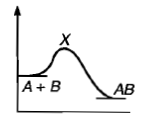2.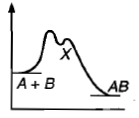3.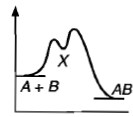4. All of the above.

Subtopic:  Arrhenius Equation |
To view explanation, please take trial in the course below.
NEET 2023 - Target Batch - Aryan Raj Singh
To view explanation, please take trial in the course below.
NEET 2023 - Target Batch - Aryan Raj Singh
Please attempt this question first.
Launched MCQ Practice Books

Prefer Books for Question Practice? Get NEETprep's Unique MCQ Books with Online Video/Text Solutions via Telegram Bot

For the non-stoichiometric reaction 2A + B $\to$ C +D, the following kinetic data were obtained in three separate experiments, all at 298 K.

 Initial  Concentration (A) Initial Concentration (B) Initial rate of formation of C (mol L-1 S-1) 0.1 M 0.1 M $1.2×{10}^{-3}$ 0.1 M 0.2 M $1.2×{10}^{-3}$ 0.2 M 0.1 M $2.4×{10}^{-3}$

The rate law for the formation of C is:

1. d[C]/dt = k[ A][B]

2. d[C]/dt = k[ A]2[B]

3. d[C]/dt = k[ A][B]2

4. d[C]/dt = k[ A]

Subtopic:  Order,Molecularity and Mechanism |
To view explanation, please take trial in the course below.
NEET 2023 - Target Batch - Aryan Raj Singh
Please attempt this question first.
Launched MCQ Practice Books

Prefer Books for Question Practice? Get NEETprep's Unique MCQ Books with Online Video/Text Solutions via Telegram Bot

For the reaction N2 + 3H2 $\to$ 2NH3, the rate $\frac{\mathrm{d}\left[{\mathrm{NH}}_{3}\right]}{\mathrm{dt}}$= 2 x 10-4 M s-1 .Therefore, the rate $\frac{-\mathrm{d}\left[{\mathrm{N}}_{2}\right]}{\mathrm{dt}}$ is given as:

1. 10-4 Ms-1

2. 104 Ms-1

3. 10-2 sM-1

4. 10-4 sM-1

Subtopic:  Definition, Rate Constant, Rate Law |
To view explanation, please take trial in the course below.
NEET 2023 - Target Batch - Aryan Raj Singh
To view explanation, please take trial in the course below.
NEET 2023 - Target Batch - Aryan Raj Singh
Please attempt this question first.
Launched MCQ Practice Books

Prefer Books for Question Practice? Get NEETprep's Unique MCQ Books with Online Video/Text Solutions via Telegram Bot

If 'I' is the intensity of absorbed light and 'c' is the concentration of AB for the photochemical process AB + hv→  AB *, the rate of formation of AB * is directly proportional to:

1. c

2. I

3. I2

4. cI

Subtopic:  Definition, Rate Constant, Rate Law |
To view explanation, please take trial in the course below.
NEET 2023 - Target Batch - Aryan Raj Singh
Please attempt this question first.
Launched MCQ Practice Books

Prefer Books for Question Practice? Get NEETprep's Unique MCQ Books with Online Video/Text Solutions via Telegram Bot

Which curve represents zero order reaction?

1.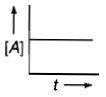2.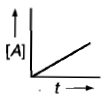3.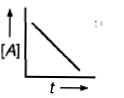4.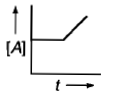Subtopic:  Order,Molecularity and Mechanism |
To view explanation, please take trial in the course below.
NEET 2023 - Target Batch - Aryan Raj Singh
Please attempt this question first.
Launched MCQ Practice Books

Prefer Books for Question Practice? Get NEETprep's Unique MCQ Books with Online Video/Text Solutions via Telegram Bot

In a reaction, the rate expression is, rate = K[A][B]2/3[C]0 , the order of reaction is:

1. 1

2. 2

3. 5/3

4. zero

Subtopic:  Order,Molecularity and Mechanism |
To view explanation, please take trial in the course below.
NEET 2023 - Target Batch - Aryan Raj Singh
Please attempt this question first.
Launched MCQ Practice Books

Prefer Books for Question Practice? Get NEETprep's Unique MCQ Books with Online Video/Text Solutions via Telegram Bot

The rate of a reaction get doubles when the temperature changes from 7°C to 17°C. By what factor will it change for the temperature change from 17°C to 27°C?

1. 1.81

2. 1.71

3. 1.91

4. 1.76

Subtopic:  Arrhenius Equation |
To view explanation, please take trial in the course below.
NEET 2023 - Target Batch - Aryan Raj Singh
Please attempt this question first.
Launched MCQ Practice Books

Prefer Books for Question Practice? Get NEETprep's Unique MCQ Books with Online Video/Text Solutions via Telegram Bot